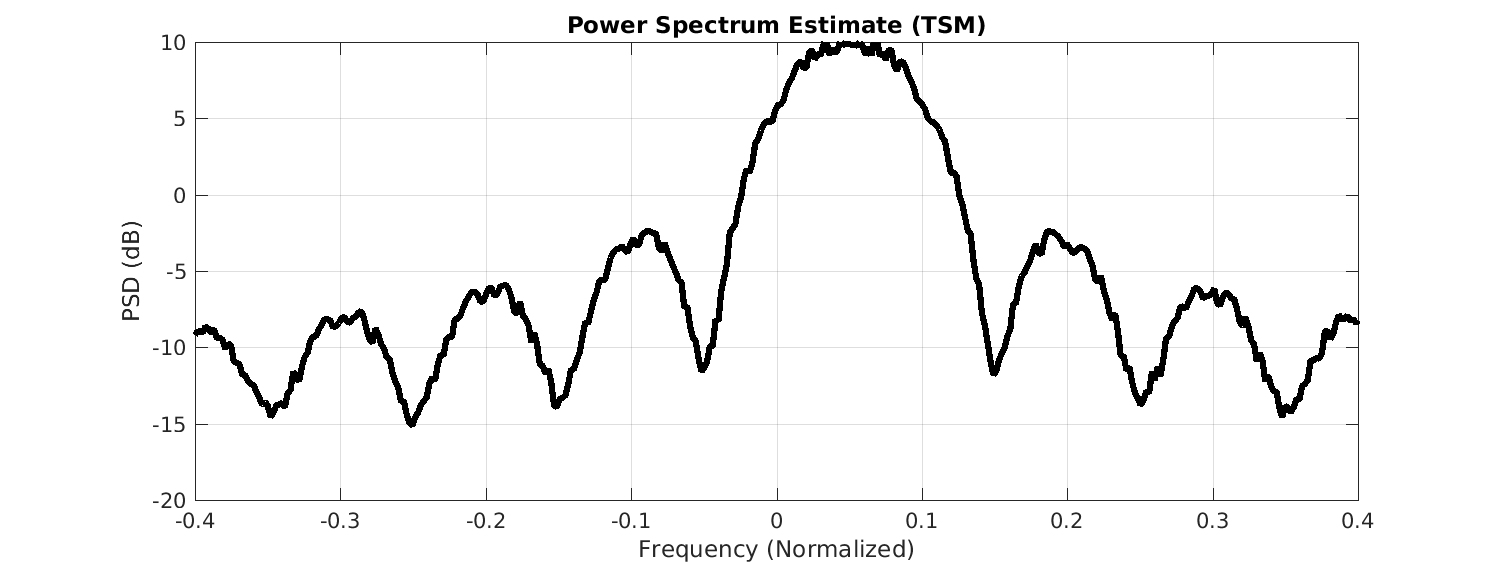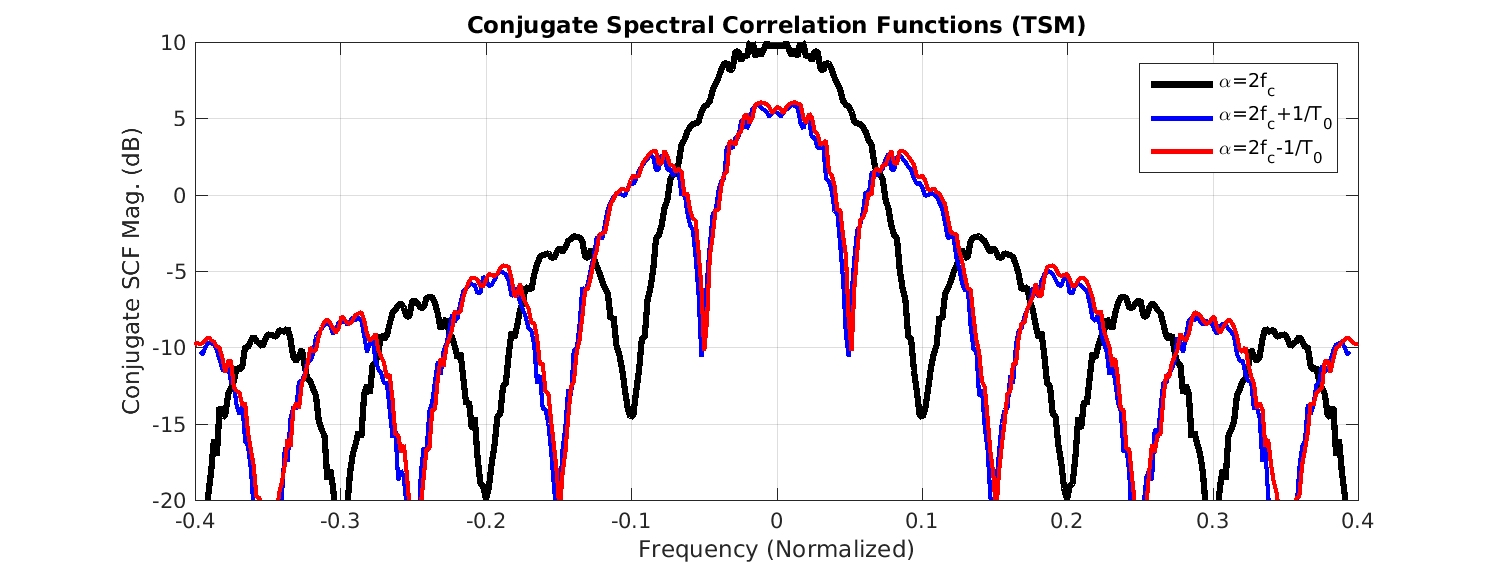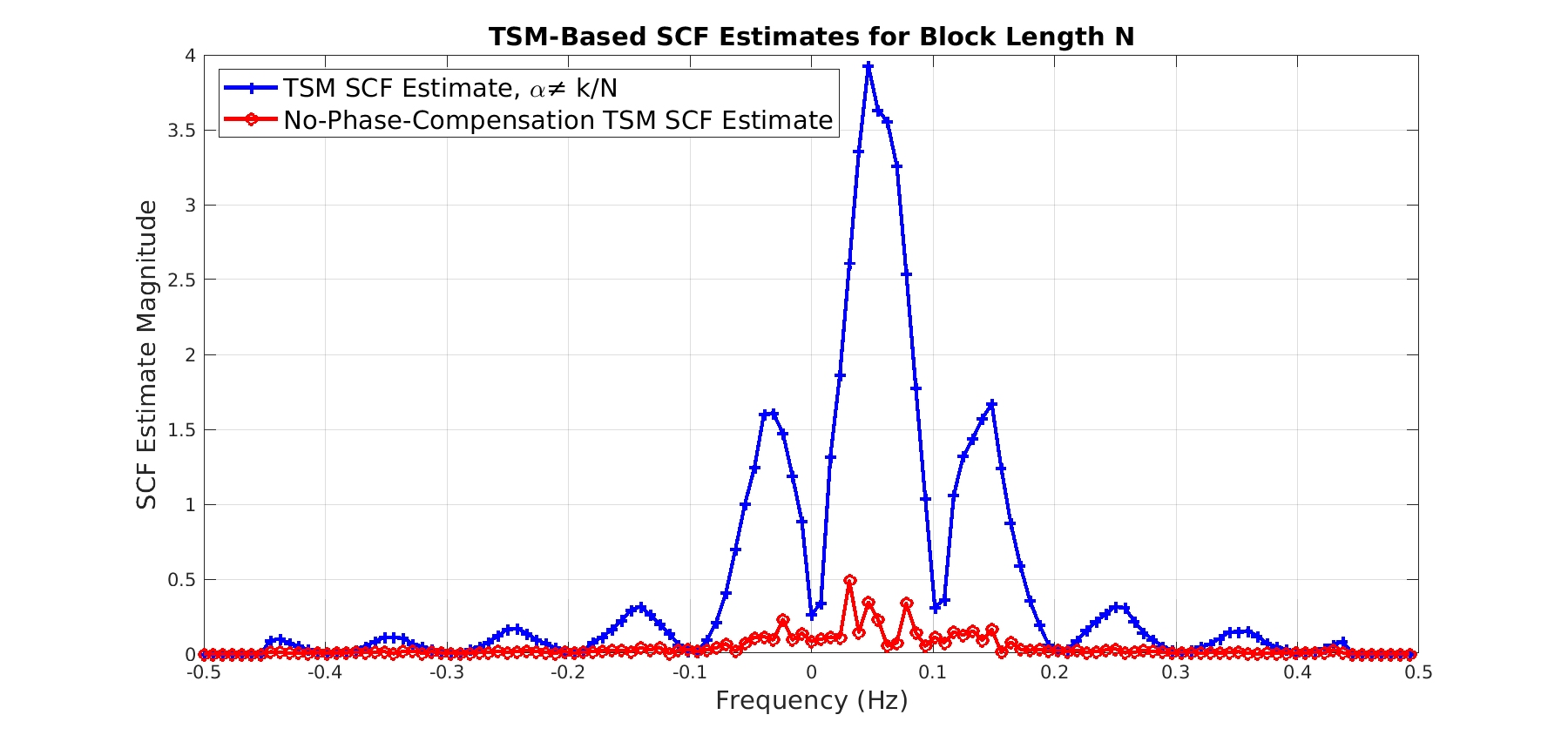# CSP Estimators: The Time Smoothing Method

In a previous post, we introduced the frequency-smoothing method (FSM) of spectral correlation function (SCF) estimation. The FSM convolves a pulse-like smoothing window$g(f)$ with the cyclic periodogram to form an estimate of the SCF. An advantage of the method is that is allows fine control over the spectral resolution of the SCF estimate through the choice of$g(f)$, but the drawbacks are that it requires a Fourier transform as long as the data-record undergoing processing, and the convolution can be expensive. However, the expense of the convolution can be mitigated by using rectangular$g(f)$.

In this post, we introduce the time-smoothing method (TSM) of SCF estimation. Instead of averaging (smoothing) the cyclic periodogram over spectral frequency, multiple cyclic periodograms are averaged over time. When the non-conjugate cycle frequency of zero is used, this method produces an estimate of the power spectral density, and is essentially the Bartlett spectrum estimation method. The TSM can be found in My Papers  (Eq. (54)), and other places in the literature.

The basic idea is to segment the provided data record into$M$ contiguous blocks of$N$ samples each, compute the cyclic periodogram for each block, and average the results. Since we will likely use the FFT to compute the Fourier transform, we will be viewing each$N$-sample block as if its time samples correspond to$t = 0, 1, \ldots, N-1$, and so the cyclic polyspectrum formula of My Papers  will have to be slightly modified to take into account the actual temporal start time for each block. This amounts to a phase compensation of each cyclic periodogram before it enters the averaging operation.

So let’s consider the Fourier transform (DFT) of a block of data that is shifted from the origin by some amount of time$u$,$X(u, f) = \displaystyle\sum_{t=0}^{N-1} x(t+u) e^{-i 2 \pi f t}. \hfill (1)$

The periodogram and cyclic periodogram are then functions of time offset$u$ as well,$I(u, f) = \displaystyle\frac{1}{N} \left| X(u, f) \right|^2, \hfill (2)$

and$I^\alpha(u, f) = \displaystyle\frac{1}{N} X(u, f+\alpha/2) X^*(u, f-\alpha/2) \hfill (3)$

and similarly for the conjugate cyclic periodogram. The TSM estimate of the SCF is simply the average value of the cyclic periodogram over all available values of$u$,$\hat{S}_x^\alpha (f) = h(u) \otimes I^\alpha(u, f), \hfill (4)$

where$h(u)$ is some pulse-like temporal window. In practice, the FFT is used to create each cyclic periodogram, so their relative phases are no longer taken into account. According to our Fourier transform result for a delayed signal, however, we can easily take this into account by multiplying each cyclic periodogram by$e^{-i 2 \pi \alpha D}$, where$D$ represents the left edge (starting point) of the subblock. For blocks having length$N$ samples, then, the value of$D$ for the$j$th block is simply$jN$. Our final TSM estimator expression is$\hat{S}_x^\alpha(f) = \displaystyle\frac{1}{M} \displaystyle\sum_{j=0}^{M-1} \left[\tilde{I}^\alpha(jN, f) e^{-i 2 \pi \alpha j N} \right], \hfill (5)$

where$\tilde{I}^\alpha(jN, f)$ is just the cyclic periodogram created from the$j$th block of$N$ samples using the FFT. Notice that when the cycle frequency is set to zero, the SCF estimate is an estimate of the PSD, and the TSM just averages$M$ periodograms, as in the Bartlett spectrum estimation method. Here is the TSM (Bartlett) PSD estimate for our rectangular-pulse BPSK signal:Figure 1. TSM-based power spectral density estimate for a rectangular-pulse BPSK signal with bit rate 1/T0=0.1 and carrier frequency offset fc=0.05. The theoretical power spectrum is a squared sinc() function.

For this PSD estimate the data-record length is$32,768$ samples and the TSM block length is$256$ samples, leading to$M = 32768/256 = 128$ blocks. Recall that the bit rate for the BPSK signal is$1/T_0 = 1/10$ and the carrier frequency is$f_c = 0.05$ (in normalized frequency units).

The TSM PSD estimate matches the FSM PSD estimate in the FSM post.

The TSM-based spectral correlation function estimates for the BPSK signal’s non-conjugate cycle frequencies are shown below:Figure 2. TSM-based non-conjugate spectral correlation function estimates for a rectangular-pulse BPSK signal with bit rate 1/T0=0.1 and carrier fc=0.05.

and the conjugate-SCF estimates are:Figure 3. TSM-based conjugate spectral correlation estimates for a rectangular-pulse BPSK signal with bit rate 1/T0=0.1 and carrier fc=0.05.

Again, these TSM estimates match quite well with the FSM estimates.

The reason the TSM and FSM estimates match so well is that the temporal and spectral resolution parameters of the estimates are similar. For both methods, the temporal resolution is equal to the data-record length ($32,768$ samples). For the FSM, the spectral resolution of the estimates is equal to the width of the frequency-smoothing window$g(f)$, and for the TSM, the spectral resolution is equal to the intrinsic spectral resolution of each cyclic periodogram, which is equal to the reciprocal of the TSM block length (in normalized units), or$1/N$.

For the FSM results in the FSM post, the spectral resolution is$0.005$ Hz$(164$ points in$g(f))$, and for the TSM results in this post, the spectral resolution is$1/256 = 0.0039$ Hz. The cycle-frequency resolutions for the two estimates are also the same at the reciprocal of the processed data-record length$\Delta\alpha \approx 1/(NM) = 1/32768$. So the two estimates have comparable time, frequency, and cycle-frequency resolution parameters, and so produce similar results. The relationship between estimator quality and the temporal, spectral, and cycle-frequency resolutions is discussed in this post.

### More on the TSM Phase-Compensation Factor

Some readers question the need for the phase-compensation factor in (5), which is given by$\displaystyle e^{-i 2 \pi \alpha j N}.$

In fact it is not needed whenever it is always equal to a constant, such as one. This happens whenever the product$\alpha j N$ is equal to an integer for all values of$j$. A sufficient condition is that the cycle frequency$\alpha$ is equal to a harmonic of$1/N$, that is,$\alpha = k/N$. However, the phase factor is needed otherwise. Here are two confirming examples (do your own when you implement the TSM).

First, let’s look at the case where the cycle frequency is just the bit rate of our usual BPSK signal, or$\alpha = 1/10$. Let’s pick the TSM block length$N=128$ and process a total of$NM = 32,768$ samples. We’ll plot the SCF estimate magnitude for the correct TSM and for the TSM without the phase-compensating factor:Figure 4. Illustration of the absolute need for the phase-compensating factor in the TSM when the cycle frequency is not a multiple of the reciprocal of the block length N.

In this case,$\alpha = 1/10 \neq k/128$ for all integers$k$, and the phase-compensating factor is clearly needed.

On the other hand, consider a BPSK signal with bit rate$1/8$ and carrier$0.05$. Choose the bit-rate cycle frequency$\alpha = 1/8$, which is in fact equal to$k/128$ for$k = 16$:Figure 5. Illustration of the lack of need for the phase-compensating factor in the TSM in the case where the cycle frequency is a multiple of the reciprocal of the block length,

And so in this case the phase-compensating factor is irrelevant since it is equal to one for all the TSM blocks, and the two TSM estimates are identical.I'm a signal processing researcher specializing in cyclostationary signal processing (CSP) for communication signals. I hope to use this blog to help others with their cyclo-projects and to learn more about how CSP is being used and extended worldwide.

Posted on

## 9 thoughts on “CSP Estimators: The Time Smoothing Method”

1.aapocketz says:

When using the TSM, you must calculate the conjugate cyclic periodogram by conjugate multiplication of the Fourier transform at offset +alpha/2 and -alpha/2.

Functionally, that seems to mean that you are multiplying the Fourier transform that has been circularly shifted left by 1 bin, by a conjugate circularly shifted right by 1 bin, to get the cyclic value at alpha = 2*Fs/N. You would circular shift by 2 bins to get alpha at 4*Fs/N, and so on all the way up to N bins, the maximum alpha shift at Fs.

Does that sound right? That means that including negative alpha values, the most alpha points will be N, and the max alpha value will be Fs, and the min alpha will therefore be 2*Fs/N?

Is there a way to get the alpha resolution (2*Fs/N) as fine as the frequency resolution (Fs/N) via some other mechanism? Do the FAM and SCCA algorithms have the same limitations on alpha?

Thank you for your blog and papers and time!

1.Chad Spooner says:

When using the TSM, you must calculate the conjugate cyclic periodogram by conjugate multiplication of the Fourier transform at offset +alpha/2 and -alpha/2.

The conjugate cyclic periodogram is used to estimate the conjugate spectral correlation function and, unfortunately, it involves no conjugations. That is, the conjugate cyclic periodogram is X(f+a/2)X(a/2-f), whereas the (non-conjugate) cyclic periodogram goes like X(f+a/2)X*(f-a/2). So that is just terminology. I’m assuming that the rest of the comment is concerned with the non-conjugate cyclic periodogram and spectral correlation function.

Functionally, that seems to mean that you are multiplying the Fourier transform that has been circularly shifted left by 1 bin, by a conjugate circularly shifted right by 1 bin, to get the cyclic value at alpha = 2*Fs/N.

Right.

That means that including negative alpha values, the most alpha points will be N, and the max alpha value will be Fs, and the min alpha will therefore be 2*Fs/N?

You must use shifts that are equal to integer numbers of the FFT bins, yes, but there is nothing preventing you from using different shifts for the two involved shifted Fourier transforms. For example, you could form X(f + 1/N)X*(f). So that is a shift of one FFT bin for X(f + a/2) and no shift for X(f – a/2). This product ends up providing an estimate of S^b(g), where b = 1/N and g = f-1/N. So the minimum cycle frequency that you can look at (besides zero) is 1/N (or in your terminology Fs/N). Agree?

Is there a way to get the alpha resolution (2*Fs/N) as fine as the frequency resolution (Fs/N) via some other mechanism?

I’m working on a post on the three different kinds of resolutions involved in CSP (temporal, spectral, and cycle), but for now I think my comment above shows that the cycle resolution is Fs/N. Yes, this is equal to the native spectral resolution of the DFT using N points, but be careful because that is not the spectral resolution of the spectral correlation measurement in general. For the TSM, where we have N total samples and the FFTs have size M << N, the spectral resolution is 1/M (Fs/M in physical Hz). For the FSM, it is the width of the spectral smoothing window, which usually contains many of the N FFT points. For the SSCA, it is the reciprocal of the number of channels in the front-end channelizer.

It turns out the cycle resolution is always about equal to the reciprocal of the total number of samples processed, whereas the spectral resolution varies by estimator and is strongly affected by estimator parameters.

2.Pablo says:

I am having trouble understanding and implementing the delay needed to estimate the cyclic periodogram from the FFT. To start with, I am not completely sure whether I understand the need for it. I take that the idea behind this is to actually estimate the band-pass zero centered signals from the FFT bins in the spectrogram, but I am not sure of why this is needed (the covariance should cancel out this delay as it is the same for the two signals). Furthermore, I’ve been testing my implementation and with/without the delay compensation results seem fairly similar (if any, the version without the delay seems slightly better). It may be that the signal length I am using is big enough to “average out” the delay effects, but I have not been able to find a case where the delay compensed option is significantly better (I take I may have an error on my own implementation).

Any insight that you can provide?

Thanks and really incredible job with this blog.

1.Chad Spooner says:

What is the TSM block length$N$ in samples, and what are the cycle frequencies$\{\alpha\}$ that you are passing to the TSM (normalized frequencies)?

Because this phase-compensating factor has historically been met with confusion and resistance, I’m working on an addendum to the TSM post… I’m hoping to be much more clear about it than I’ve been in the past. Sorry about that!

1.Pablo says:

I’ve been playing around with different values and signals. Using your BPSK signal and the values given in the post (N = 256, etc), I’ve been able to reconstruct something similar to your plots, both with no phase-compensation and with my maybe incorrect one. With respect to the cycle frequencies, I am generating the complete square matrix (every combination of spectral correlation). For the delay compensation I am using normalized frequencies (alpha = [0, 1/256, 2/256, … 255/256]) and multiples of N for D, i.e: [0, 256, 512, …] up to the last segment. I understand that this delay does not change between spectral components (FFT bins). Not sure if this gives you an idea of where my mistake is. If you have any reference I can use to better understand the phase compensation, I am willing to read it.

Even with my likely ill-formed SCF I have been able to see some interesting features of different modulations, this is a really interesting topic.

Thanks a lot!

1.Chad Spooner says:

Let’s say you are processing a total of$T$ samples, and your TSM block size is$N$ samples. Recalling the basics of cycle-frequency resolution, cycle frequencies separated by about$1/T$ will be distinguishable. So a full spectral correlation analysis must consider the cycle frequencies$k/T$,$k = 0, 1, 2, \ldots T-1$. If you consider instead the set of cycle frequencies$k/N$,$k = 0, 1, 2, \ldots N-1$, you will be missing a lot of cycle frequencies (more and more as$T/N$ increases with$N$ fixed). In either case, the phase-compensating factor will be one for all cycle frequencies and all block indices if both$T$ and$N$ are dyadic (powers of two).

More to the point, what happens with your two versions (one with my phase correction, one without) when you use my rectangular-pulse BPSK signal as an input and you specify the single cycle frequency$1/10$ (which is the bit rate of the rectangular-pulse BPSK signal)? Here the cycle frequency is not the reciprocal of a dyadic number. For my TSM implementations, only the one with the phase-compensator works.

1.Pablo says:

I now see where my mistake was. I thought I was using N=256, but actually, I was using N=100, so 1/10 was one of the values generated. This way, the delay compensation plays no role, and so the estimation held up without delay compensation. Changing to N=256, the delay compensation is indeed needed and works as expected.

Thanks a lot for your support. I will keep on digging in these concepts!

3.Masoud Farshchian says:

Quick question: 1) “The spectral resolution is 0.005 Hz (164 points in g(f))”.. would it actually be 1/164 which is 0.0061 or do you take the RMS bandwidth which in the case of rectangular window would match the support?

Thanks

1.Chad Spooner says:

Masoud: Thanks for visiting the CSP Blog and leaving a comment!

In the FSM post example, I mentioned that the processing block length is 32768 samples. That means each FFT bin would represent 1/32768 Hz (normalized frequencies here). And therefore the samples in the cyclic periodogram are separated by that same amount, 1/32768 Hz. If the smoothing window width is 164 frequency samples, the spectral resolution of the smoothed cyclic periodogram is therefore 164/32768 = 0.005 Hz. I also mention using zero-padding in the FSM post. To maintain an effective spectral resolution of 0.005 Hz, the number of points in the smoothing window in that case is 328 because the FFT bin width is then 1/65536.